# Channel with a finite memory

(diff) ← Older revision | Latest revision (diff) | Newer revision → (diff)

A communication channel for which the statistical properties of the output signal at a timeare determined by the input signals transmitted at the times,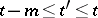(and therefore do not depend on the signals transmitted prior to the time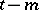); the numberis called the size (or length) of the memory of the channel.

More precisely, a discrete-time communication channel where the input and output signals are given, respectively, by random sequences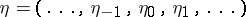andwith values in the spaces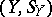andis called a channel with a finite memory if a compatible set of conditional distributions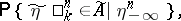by means of which such a channel can be defined, satisfies for any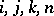, and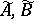the conditions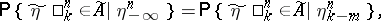Here,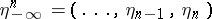, and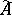(respectively,) is a set in the direct product of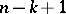(respectively,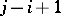) copies of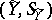. A continuous-time channel with a finite memory is defined similarly.

How to Cite This Entry:
Channel with a finite memory. Encyclopedia of Mathematics. URL: http://encyclopediaofmath.org/index.php?title=Channel_with_a_finite_memory&oldid=11735
This article was adapted from an original article by R.L. DobrushinV.V. Prelov (originator), which appeared in Encyclopedia of Mathematics - ISBN 1402006098. See original article